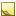﻿Functions in templatesFunctions in templates

When you write template files, you can make function calls to transform the data from the input into a form you want to use in the output. There are two types of functions you can call from within a template:

• Built-in functions (specific to TDE):
• Comparison functions (for use with [Filter] only)
• Accumulator functions (for use with GroupBy only)
• Data transformation functions
• .NET functions

This topic contains the following sections:Built-in functions: Comparison functions

Comparison functions are only used inside the Filter section of a template, and indicate the subset of values that are to be used. For details, see [Filter] section.Built-in functions: Accumulator functions

Accumulator functions are supported only if the Template section includes a GroupBy entry. They let you summarize all of the rows in a group on columns that are not the grouping column(s).Note

Recommended: In templates that use a GroupBy entry in the Template section, summarize all columns (other than the grouping columns) using accumulator functions. Any output values that are not generated using an accumulator function are based on one of the rows within the group, but which row is arbitrary. That means that, if the column does not have the same value for all rows in the group, the resulting output value is not well-defined.

The following table lists all of the accumulator functions:

FunctionDescription
Sum(Number)Sums the numeric values over all input rows in a single group from the input file.
Min(Number or Alphabetic)Returns the minimum value within the group of input rows.
Max(Number or Alphabetic)Returns the maximum value within the group of input rows.
Count(Any type)Returns the total number of input rows in the group.
Collect(String or Enumerable data) Generates an aggregate value for the values in the input rows of the group. If the input values are strings, the result is a comma-delimited string. Otherwise, the result is an array of values.Built-in functions: Data transformation functions

Data transformation functions can be used anywhere within a template. Following are all of the data transformation functions:

FunctionDescription
ExtractDate(Timestamp)Extracts the date from a Timestamp variable. Returns a new Timestamp.
ExtractTime(Timestamp)Extracts the time from a Timestamp variable. Returns a Text value in the format of HH:mm:ss.
AccumulateOdometer(Latitude, Longitude, Tag)Sums the distance between points until the Tag changes. Latitude and Longitude are Angles, Tag is a Text value. Returns a Distance value.
Join(Array, Text)Formats a string representing the elements of an array, separated by a specified separator character. Returns a Text value.
Id(string)Generates an Id value from a string input.
RenderXml(xml)Only valid for XPath or JSON input. Renders the full XML text of all the nodes selected by the XPath query. Returns a Text value.
ParseXml(string)Only valid for XPath or JSON output. Parses the string as XML which is then inserted directly into the output file. Returns a Text value.

Deprecated data transformation functions

The following data transformation functions have been deprecated. They will still work if you use one of them in a template, but you can now access this data natively within a template using subfield syntax (for example, Address.City). For more information about working with subfields, see Accessing subfields.

FunctionDescription
ExtractLat(Location)Extracts the latitude from a Location. Returns an Angle value.
ExtractLon(Location)Extracts the longitude from a Location. Returns an Angle value..NET functions

TDE provides an infrastructure that lets you make use of .NET functions (methods). It does this by converting built-in data types to the corresponding .NET types and then matching the arguments to the specified function and class.Note

Currently, .NET String, DateTime, and TimeSpan functions are supported. Extension to other .NET functions may be added in future versions.

.NET Examples: TimeSpan, DateTime methods

These template calculations achieve the same goal, which is to subtract 3 hours from the input in the LocalTime column. The first one shows usage of both TimeSpan and DateTime methods:

```[Calculated]
TimeZoneOffset(TimeInterval) = TimeSpan.Negate(TimeSpan.FromHours(3))
LocalTime(Timestamp) = Input.LocalTime

This version uses only the DateTime function:

```[Calculated]

#### Syntax

.NET functions are invoked in a template using syntax similar to that of calling a static function in a .NET program.

Static functions

When calling a .NET static function, the syntax is exactly like calling a static function in a .NET program. Example:

`A = String.IsNullOrWhiteSpace(Input.Id)`

Instance functions

When calling a .NET instance function, the syntax is changed to look like a static function, with the object instance passed in as the first argument. Examples:

```B = String.Trim(Input.Id)                  # equivalent to Input.Id.Trim() in .NET
C = String.TrimStart(Input.Id,["{","~"])   # equivalent to Input.Id.TrimStart(new[] {'{', '~'}) in .NET, which trims one or more characters
D = String.PadLeft(Input.Id, 12, "0")      # equivalent to Input.Id.PadLeft(12, 0) in .NET```

Restrictions

• Functions that take ref or out parameters are not supported.
• Functions that take a params array argument do not support a variable number of arguments. Instead, you must explicitly pass in an array literal. Example:
`D = String.Format("Id: {0}, Tag: {1}", [Input.Id, Input.Tag])    # Do NOT use String.Format("Id: {0}, Tag: {1}", Input.Id, Input.Tag)`